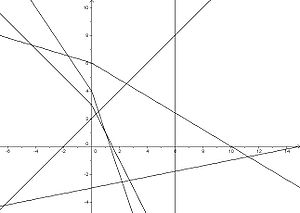# Moulton plane

﻿
Moulton planeThe Moulton plane. Lines sloping down and to the right are bent where they cross the y-axis.

In incidence geometry, the Moulton plane is an example of an affine plane in which Desargues' theorem does not hold. It is named after the American astronomer Forest Ray Moulton. The points of the Moulton plane are simply the points in the real plane R2 and the lines are the regular lines as well with the exception, that for lines with a negative slope the slope doubles when they pass the y-axis.

## Formal definition

The Moulton plane is an incidence structure$\mathfrak M=\langle P, G,\textrm I\rangle$, where P denotes the set of points, G the set of lines and I the incidence relation "lies on":$P:=\mathbb R^2 \,$$G:=(\mathbb R \cup \{\infty\}) \times \mathbb R,$$\infty$ is just a formal symbol for an element$\not\in\mathbb R$. It is used to describe vertical lines, which you may think of as lines with an infinitely large slope.

The incidence relation is defined as follows:

For p = (xy) ∈ P and g = (mb) ∈ G we have$p\,\textrm I\,g\iff\begin{cases} x=b&\text{if }m=\infty\\ y=mx+b&\text{if }m\geq 0\\ y=mx+b&\text{if }m\leq 0, x\leq 0\\ y=2mx+b&\text{if }m\leq 0, x\geq 0. \end{cases}$

## Application

The Moulton plane shows that affine planes in which Desargues' theorem does not hold do actually exist. As a consequence, the associated projective plane into which an affine plane can be extended, is non-desarguesian as well. Since in PG(2,F) Desargues' theorem does hold, this means that there exist projective planes that are not isomorphic to PG(2,F). In other words not all projective planes can be described via the canonical construction (P(V)) over a 3-dimensional vector space V.